SSC  >  Quantitative Aptitude - Test 10

# Quantitative Aptitude - Test 10

Test Description

## 25 Questions MCQ Test SSC CGL Tier 1 Mock Test Series | Quantitative Aptitude - Test 10

Quantitative Aptitude - Test 10 for SSC 2022 is part of SSC CGL Tier 1 Mock Test Series preparation. The Quantitative Aptitude - Test 10 questions and answers have been prepared according to the SSC exam syllabus.The Quantitative Aptitude - Test 10 MCQs are made for SSC 2022 Exam. Find important definitions, questions, notes, meanings, examples, exercises, MCQs and online tests for Quantitative Aptitude - Test 10 below.
Solutions of Quantitative Aptitude - Test 10 questions in English are available as part of our SSC CGL Tier 1 Mock Test Series for SSC & Quantitative Aptitude - Test 10 solutions in Hindi for SSC CGL Tier 1 Mock Test Series course. Download more important topics, notes, lectures and mock test series for SSC Exam by signing up for free. Attempt Quantitative Aptitude - Test 10 | 25 questions in 15 minutes | Mock test for SSC preparation | Free important questions MCQ to study SSC CGL Tier 1 Mock Test Series for SSC Exam | Download free PDF with solutions
 1 Crore+ students have signed up on EduRev. Have you?
Quantitative Aptitude - Test 10 - Question 1

### A cistern 5 m long and 8 m wide, contains water up to depth of 2 m 45 cm. Find the total area of wet surface.

Detailed Solution for Quantitative Aptitude - Test 10 - Question 1

A cistern 5 m long and 8 m wide, contains water up to depth of 2 m 45 cm.
Here, cistern is in cuboid form
Length of cistern = 5 m
Breadth of cistern = 8 m
Height of cistern upto which its wet = 2 m 45 cm = 2.45 m
Total area of wet surface = {2(length + breadth) × height} + (length × breadth)
= {2(5 + 8) × 2.45} + (5 × 8)
= 63.7 + 40
=103.7 m2

Quantitative Aptitude - Test 10 - Question 2

### In a triangle ABC, ∠B = 90°, D is a point on line AB such that it divides AB into two equal parts. AB is denoted by 2x and AC by y, then find the value of sin θ where ∠DCB = θ°.

Detailed Solution for Quantitative Aptitude - Test 10 - Question 2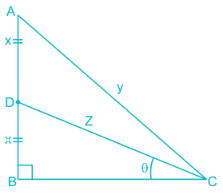Appling Pythagoras theorem on triangle ABC
AC2 = AB2 + BC2
⇒ y2 = (2x)2 + BC2
⇒ BC2 = y2 – 4x2
Now applying Pythagoras theorem on triangle DBC
DC2 = DB2 + BC2
⇒ z2 = x2 + BC2
⇒ BC2 = z2 – x2
Now, on comparing the value of BC2 we get
y2 – 4x2 = z2 – x2
∴ z2 = y2 – 3x2

∴ z = √(y2 – 3x2)
Now in Triangle DBC,
sin θ = DB/DC
∴ sin θ = x/z
∴ sin θ = x/√(y2 – 3x2)

Quantitative Aptitude - Test 10 - Question 3

### The area of a circle is proportional to the square of its radius. A small circle of radius 3 cm is drawn within a larger circle of radius 5 cm. find the ratio of the area of the annular zone to the area of the larger circle. (Area of the annular zone is the difference between the area of the larger circle and that of the smaller circle).

Detailed Solution for Quantitative Aptitude - Test 10 - Question 3

Area of a circle = π(radius)2
A small circle of radius 3 cm is drawn within a larger circle of radius 5 cm.
Area of large circle = π(5)2
= (22/7) × (5)2
= (22 × 5 ×5)/7
= 550/7
Area of small circle = π (3)2
= (22 × 3 × 3)/7
= 198/7
Area of annular zone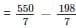= 352/7
The ratio of the area of the annular zone to the area of the larger circle = area of annular zone: area of large circle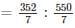= 16: 25

Quantitative Aptitude - Test 10 - Question 4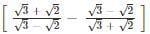simplifies to

Detailed Solution for Quantitative Aptitude - Test 10 - Question 4

Given expression is,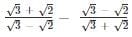Rationalising, we get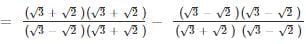Using identity: a2 – b2 = (a – b)(a + b) and a2 + b2 = a2 + 2ab + b2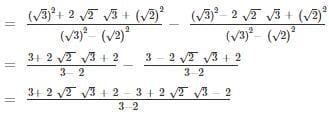= 4 × √2 × √3
= 4√6

Quantitative Aptitude - Test 10 - Question 5

If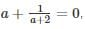then the value of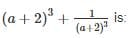Detailed Solution for Quantitative Aptitude - Test 10 - Question 5

The given expression is: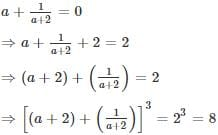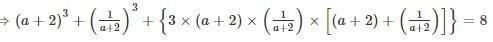[Using the formula (a + b)= a+ b+ 3ab(a + b)]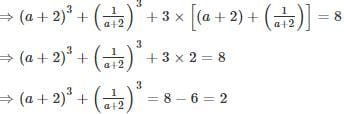Trick:
(a + 2) + 1/(a + 2) = 2
If x + 1/x = k then the value of x3 + 1/x3 will always be equal to (k3 – 3k)
Here k = 2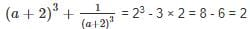Quantitative Aptitude - Test 10 - Question 6

Directions: The production figure of a perfume manufactures are given in the form of percentage sub-divided bar diagram. Study the diagram and answer questions.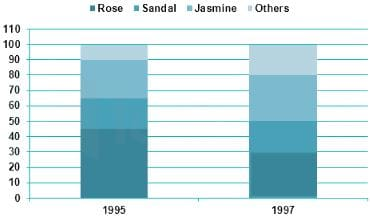Q. What is the production of jasmine perfume in the year 1997? Given that during the year 1997 total perfumed production was 5000 units.

Detailed Solution for Quantitative Aptitude - Test 10 - Question 6

Given that during the year 1997 total perfumed production was 5000 units.
From the pie chart we can see, the percentage production of jasmine perfume in the year 1997 = (80 – 50) = 30% of total production.
∴ The production of jasmine perfume in the year 1997
= 30% of 5000
= 1500
∴ The production of jasmine perfume in the year 1997 = 1500

Quantitative Aptitude - Test 10 - Question 7

Directions: The production figure of a perfume manufactures are given in the form of percentage sub-divided bar diagram. Study the diagram and answer questions.Q. What is the ratio of percentage production of rose perfume during 1995 to that during the year 1997?

Detailed Solution for Quantitative Aptitude - Test 10 - Question 7

From the pie chart we can see, the percentage production of rose perfume in the year 1995 = (45 – 0) = 45% of total production.
Similarly, from the pie chart we can see, the percentage production of rose perfume in the year 1997 = (30 – 0) = 30% of total production.
The ratio of percentage production of rose perfume during 1995 to that during the year 1997 = 45: 30 = 3 : 2

Quantitative Aptitude - Test 10 - Question 8

Directions: The production figure of a perfume manufactures are given in the form of percentage sub-divided bar diagram. Study the diagram and answer questions.Q. What is the percentage increase of % production of sandal perfume during the year 1995 over that during 1997?

Detailed Solution for Quantitative Aptitude - Test 10 - Question 8

The percentage of production of sandal perfume during the year 1995 = (65 – 45) = 20%
The percentage of production of sandal perfume during the year 1997 = (50 – 30) = 20%
∴The percentage of production of sandal perfume during the year 1995 over that during 1997 = 0%

Quantitative Aptitude - Test 10 - Question 9

From a cliff 120 m above the shore of a sea, the angle of depression of a ship is 60°. Find the distance from the ship to a point on the shore directly below the observer.

Detailed Solution for Quantitative Aptitude - Test 10 - Question 9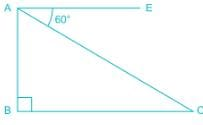From the diagram,
AB = height of the cliff = 120 m
Angle of depression = ∠ CAE = 60°
BC = Distance of the ship to the bottom of the cliff
∴ ∠ CAB = 90° - 60° = 30°
From ΔABC
tan ∠CAB = perpendicular/Base
⇒ tan 30° = BC/AB
⇒ 1/√3 = BC/120
⇒ BC = 120/√3 m
⇒ BC = 120√3/3 m = 40√3 m

Quantitative Aptitude - Test 10 - Question 10

x = m sec α cos β, y = n sec α sin β , z = p tan α , then the value of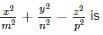Detailed Solution for Quantitative Aptitude - Test 10 - Question 10

Given that,
x = m sec α cos β
Squaring both side we can write,
x2 = m2 sec2α × cos2 β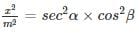Similarly we can write,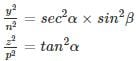Now we have to calculate the value of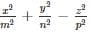Putting the values: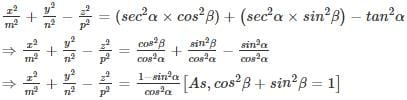Quantitative Aptitude - Test 10 - Question 11

Sin232° + sin258° + cot258° is equal to

Detailed Solution for Quantitative Aptitude - Test 10 - Question 11

Sinθ = cos(90° - θ) and sin2θ + cos2θ = 1 and Sec2θ = 1 + tan2θ
Sin232° + sin258° + cot258°
= sin232° + cos232° + cot258°
= 1 + cot258°
= cosec258°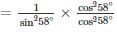= cot258°/cos258°
= cot258°/sin232°
= cosec232°cot258°

Quantitative Aptitude - Test 10 - Question 12

If the arcs of same length in two circles subtend angles of 60° and 75° at their centres, the ratio of their radii is

Detailed Solution for Quantitative Aptitude - Test 10 - Question 12

First circle subtend angle = 60°
2nd circle subtend angle = 75°
For the first circle:
⇒ angle1 = 60 = 60 × π/180 = π/3 radians
For the second circle:
⇒ angle2 = 75 = 75 × π/180 = 5 π/12 radians
We have the formula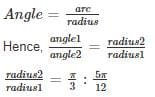Quantitative Aptitude - Test 10 - Question 13

In Δ ABC, ∠A + ∠B = 65°, ∠B + ∠C = 140°, then find ∠B.

Detailed Solution for Quantitative Aptitude - Test 10 - Question 13

We know that the sum of angles of a triangle is 180°.
∴ ∠A + ∠B + ∠C = 180°
Given: ∠A + ∠B = 65°
Replacing this value we get,
⇒ 65 + ∠C = 180
⇒∠C = 115°
Also, ∠B + ∠C = 140° (given)
⇒∠B + 115° = 140°
∴ ∠B = 25°

Quantitative Aptitude - Test 10 - Question 14

If S is the circumcentre of ΔABC and ∠A = 50°, then the value of ∠BCS is

Detailed Solution for Quantitative Aptitude - Test 10 - Question 14

Property of circumcentre
In any ΔXYZ, if O be circumcentre(where perpendicular bisectors of all three sides meet) of ΔXYZ.
Then, ∠XOZ = 2∠Y
∠ZOY = 2∠X
∠YOX = 2∠Z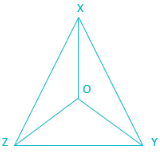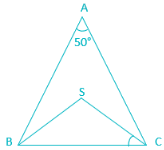Now, in ΔABC
S is the circumcentre of ΔABC and ∠A = 50°
∴ ∠BSC = 2∠A
= 2 × 50°
= 100°
Now in ΔBSC,
∠BCS = ∠CSB (as, BS and CS are radius of the circumcentre and angles opposite to the equal sides are equal)
∵ Sum of all angles of a triangle = 180°
∴ ∠BSC + ∠BCS + ∠CSB = 180°
⇒ 100° + 2∠BCS = 180°
⇒ 2∠BCS = 80°
⇒∠BCS = 40°

Quantitative Aptitude - Test 10 - Question 15

A cistern is provided with two pipes A and B. A can fill it in 20 minutes and B can empty it in 30 minutes. If A and B be kept open alternately for one minute each, how soon will the cistern be filled?

Detailed Solution for Quantitative Aptitude - Test 10 - Question 15

Given, A can fill it in 20 minutes and B can empty it in 30 minutes
Pipe A's work for one minute=1/20
Pipe B's work for one minute=1/30
∴ Total work done in two minute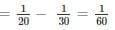In every 2 minute, 1/60th work is done, which means upto 114th minute, amount of work done will be = 57 × 1/60 = 57/60
Now the remaining work i.e. 3/60 (=1/20th) will be done by A in next 1 minute.
∴ Total time required = 114 + 1 = 115 minutes

Quantitative Aptitude - Test 10 - Question 16

A cyclist completes a certain journey in 5 hours. He covers half the distance at 16 km./hr. and the second half of the distance at 24 km./hr. The distance (in km) covered by the cyclist is?

Detailed Solution for Quantitative Aptitude - Test 10 - Question 16

Let,
Total distance covered = 2x km
We know that,
Time required = Distance covered/Speed
Given,
Half of the distance i.e. x km is covered at speed 16 km/hr
∴ Time required = x/16 hours
Next half of the distance i.e. x km is covered at speed 24 km/hr
∴ Time required = x/24 hours
Given,
Total time required = 5 hours
∴ x/16 + x/24 = 5
⇒ (3x + 2x)/48 = 5
⇒ 5x = 48 × 5
⇒ x = 48 km
∴ Total distance covered = 2 × 48 km = 96 km

Quantitative Aptitude - Test 10 - Question 17

Ram bought 1600 eggs at the rate of Rs. 3.75 per dozen. He sold 900 of them at 2 eggs for Rs. 1 and the remaining at 5 eggs for Rs. 2. Find his loss or gain per cent.

Detailed Solution for Quantitative Aptitude - Test 10 - Question 17

∵ Price of 12 eggs = Rs. 3.75
∵ Price of 1 egg = 3.75/12 = Rs.0.3125
∵ Price of 1600 eggs = Rs. (0.3125 × 1600) = Rs.500
∵ cost price, CP of eggs = Rs. 500
Selling price of 2 eggs = Rs. 1
∵ Selling price of 900 eggs =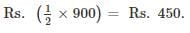Selling price of 5 eggs = Rs. 2
∴ Selling price of remaining (1600 - 900 = 700) eggs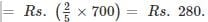∴ Total selling price, SP of eggs = Rs. 450 + 280 = Rs.730.
∵ SP > CP
∴ shopkeeper will have gain
Gain = SP – CP = Rs. 730 - 500 = Rs. 230.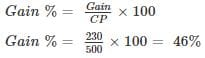Hence, Ram will have a gain percent of 46% by selling eggs.

Quantitative Aptitude - Test 10 - Question 18

A 24 litres mixture contains milk and water in the ratio 5: 1. If 4 litres of water is mixed in the mixture, the percentage of milk in the new mixture is –

Detailed Solution for Quantitative Aptitude - Test 10 - Question 18

Given,
Ratio of milk and water is 5: 1
Amount of mixture = 24 litres
∴ Amount of milk in the mixture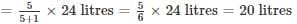∴ Amount of water in the mixture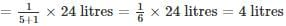If 4 litres of water is added
Amount of water in the new mixture = 4 + 4 = 8 litres
Total amount of mixture = 24 + 4 = 28 litres
And, Amount of milk in the new mixture = 20 litres
∴ Required percent
= (amount of milk/total amount in mixture) × 100%
= (20/28) × 100
= 500/7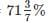Quantitative Aptitude - Test 10 - Question 19

The average age of 11 players of a cricket team is increased by 2 months when two of them aged 18 years and 20 years are replaced by two new players. The average age of the new players is

Detailed Solution for Quantitative Aptitude - Test 10 - Question 19

Formula:-
Sum of observations = average × number of observations
The average age of 11 players of a cricket team is increased by 2 months when two of them aged 18 years and 20 years are replaced by two new players
∴ Total age of players who are replaced= (18 +20) months
= 38 years
Age of 11 players of a cricket team is increased by (2 × 11= 22) months
Total Age of new players = 38years + 22 months
Average age of new players = (total age /no of players)
= (38years + 22 months)/2
= 19years 11 months

Quantitative Aptitude - Test 10 - Question 20

The value of a machine depreciates every year at the rate of 10% on its value at the beginning of the year. If the current value of the machine is Rs.729, its worth 3 years ago was.

Detailed Solution for Quantitative Aptitude - Test 10 - Question 20

Let, x be the value of the machine 3 years ago
Since, the value of machine depreciates at the rate 10% every year
∴ its value after 1 year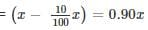Its value after 2 years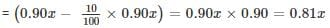Its value after 3 years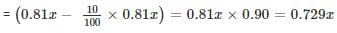According to the question,
0.729x = 729
x = 729/0.729 = 1000
Alternate:-
As we know that, if the value of machine depreciates by R% every year, then the value of machine after ‘t’ years will be:-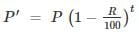Here, P’ = future value of machine
P = current value.
Here it is given that, current value is Rs. 729 and we will have to find out the value 3 years ago.
So, here P’ = 729 and P = ?, R = 10%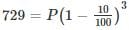⇒ P = 1000

Quantitative Aptitude - Test 10 - Question 21

Simple interest on a certain sum is 16/25 of the sum. The rate per cent if the rate per cent and time (in years) are equal, is

Detailed Solution for Quantitative Aptitude - Test 10 - Question 21

We know that, formula for simple interest: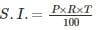Where,
S.I. = Simple Interest
P = principal
R = Rate of interest
T = Time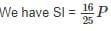Let R = T = k
Hence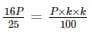1600 = 25 × k × k
64 = k2
k = +8 or k = -8
Since time period cannot be negative, hence, we have
k or rate of interest = 8 %

Quantitative Aptitude - Test 10 - Question 22

The cost of a piece of diamond varies with the square of its weight. A diamond of Rs. 5,184 value is cut into 3 pieces whose weights are in the ratio 1 : 2 : 3. Find the loss involved in the cutting.

Detailed Solution for Quantitative Aptitude - Test 10 - Question 22

Given, the cost of a piece of diamond varies with the square of its weight.
⇒ Price = k × weight2   (k is any constant)
Let the weight of a piece of diamond be ‘6x’.
Then, original price = k (6x)2 = 36kx2
⇒ 36kx2 = 5184      ……… eq(1)
The piece is cut into 3 pieces of weights ratio 1 : 2 : 3.
Now, the new price = k (x2 + (2x)2 + (3x)2) = 14kx2
From eq(1)
New price = (14 × 5184)/36 = 2016.
∴ The loss = 5184 – 2016 = Rs. 3168.

Quantitative Aptitude - Test 10 - Question 23

Simplified value of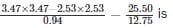Detailed Solution for Quantitative Aptitude - Test 10 - Question 23

Follow BODMAS rule to solve this question, as per the order is given below,
Step - 1 - Parts of an equation enclosed in 'Brackets' must be solved first,
Step - 2 - Any mathematical 'Of' or 'Exponent' must be solved next,
Step - 3 - Next, the parts of the equation that contains 'Division' and 'Multiplication' are calculated,
Step - 4 - Last but not least, the parts of the equation that contains 'Addition' and 'Subtraction' should be calculated.
We know that,
(a2 – b2) = (a + b)(a – b)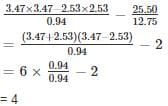Quantitative Aptitude - Test 10 - Question 24

Convert 4.327327327.... into a rational expression.

Detailed Solution for Quantitative Aptitude - Test 10 - Question 24

Let x = 4.327327327…. ………(1)
Multiply both sides by 1000
⇒ 1000x = 4327.327327… …….(2)
Subtract eq. (1) from eq. (2)
⇒ 999x = 4323
⇒ x = 4323/999

Quantitative Aptitude - Test 10 - Question 25

461 + 462 + 463 + 464 is divisible by

Detailed Solution for Quantitative Aptitude - Test 10 - Question 25

⇒ 461 + 462 + 463 + 464=461 (1 + 4 + 42 + 43)
⇒ 461 + 462 + 463 + 464=461 × 85
⇒ 461 + 462 + 463 + 464=461 × 5 × 17
So, the expression is divisible by 17

## SSC CGL Tier 1 Mock Test Series

42 docs|103 tests
 Use Code STAYHOME200 and get INR 200 additional OFF Use Coupon Code
Information about Quantitative Aptitude - Test 10 Page
In this test you can find the Exam questions for Quantitative Aptitude - Test 10 solved & explained in the simplest way possible. Besides giving Questions and answers for Quantitative Aptitude - Test 10, EduRev gives you an ample number of Online tests for practice

## SSC CGL Tier 1 Mock Test Series

42 docs|103 tests